Aptitude Tests 4 Me

Non Verbal Logic

Detailed solution

52.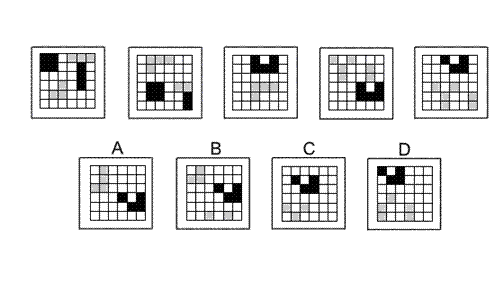Solution: A Explanation: In this question there is a grid of squares. There is a single rule to follow - each time the squares of the grid are translated 1 square to the right and three squares down. Following this rule, the next diagram of the sequence must be A.

53.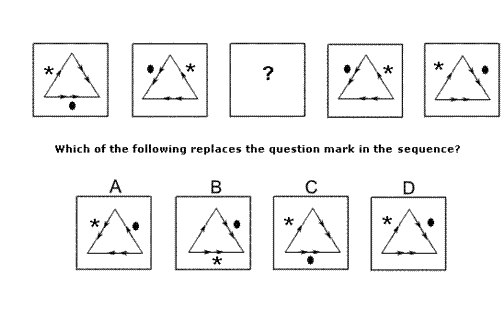Solution: D Explanation: In this question there are two rules to follow. The fist rule is that the equilateral triangle and its arrows are reflected in the vertical axis from each diagram to the next. Following this rule, the missing diagram of the sequence could be B, Cor D. The second rule is that the asterisk and the dot move around the outside of the triangle by a number of places equal to, and direction given by, the arrowheads from the previous diagram. Following this rule, the dot will move to the right edge of the triangle and the asterisk will move to the left edge – the missing diagram of the sequence, therefore, is D.

54.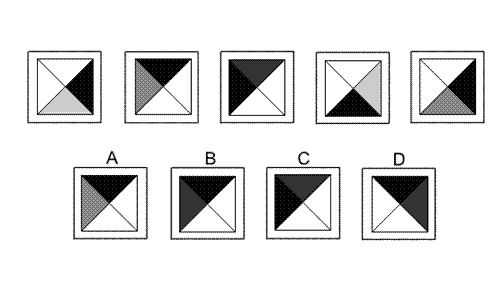Solution: B Explanation: In this question, there is a black triangle and a grey triangle which each has its own rule. The black triangle always rotates by 90° anticlockwise. Following this rule, for the next diagram in the sequence, the black triangle should be in the top quadrant of the diagram. The correct answer, therefore, could be A, B or D. The grey triangle also rotates, but by 90° clockwise each time. However, the shade of the greytriangle also changes from light grey to medium grey to dark grey, back to light grey again. Following this rule, for the next diagram of the sequence, the grey triangle should be in the left quadrant of the diagram and should be dark grey. The correct answer, therefore, is B.

55.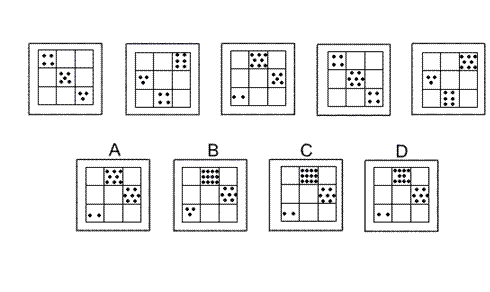Solution: C Explanation: In this question there are two rules that apply to squares with dots as they move down the columns of the grid. First rule: The squares in the first and second rows of the grid move down one place and the number of dots decreases by one. Following this rule, for the next diagram of the sequence, there should be two dots in the first column, third row and seven dots in the third column, second row. The correct answer, therefore, could be A, C or D. Second rule: The squares in the bottom row of the grid move to the top row and the numbers of dots are doubled. Following this rule, for the next diagram in the sequence, there should be twelve dots in the first row, second column. The correct answer, therefore, is C.

56.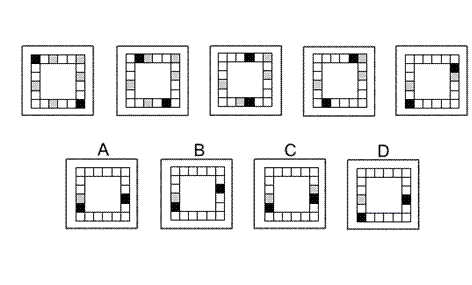Solution: A Explanation: In this question the black squares move clockwise around the perimeter of the grid. They move one place only, except when they catch up with a grey square, in which case in the next move the black square jumps over and 'takes' the grey square - just like in draughts - and the grey square is removed from the grid. Following this rule, for the next diagram in the sequence, the black square on the left will move one place, but the black square on the right will 'take' the grey square below it and move down two places. The correct answer, therefore, is A.

 1 2 3 4 5 6 7 8 9 10 11 12 13 14 15 16 17 18 19 20 21 22 23 24 25 26 27 28 29 30 31 32 33 34 35 36 37 38 39 40 41 42 43 44 45 46 47 48 49 50 51 52 53 54 55 56 57 58 59 60 61 62 63 64 65 66 67 68 69 70 71 72 73 74 75 76 77 78 79 80 81 82 83 84 85 86 87 88Passage ReadingVerbal LogicNon Verbal LogicNumerical LogicData InterpretationReasoningAnalytical AbilityBasic NumeracyAbout UsContactPrivacy PolicyMajor TestsFAQ